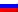UDC 37.02

CARRYING OUT TECHNIQUE CONTENT-ANALYSIS SCHOOL TEXTBOOKS OF PHYSICS AND ITS RESULTS

Mayer Robert Valerievich
FSBEI of HPE “The Glazov Korolenko State Pedagogical Institute”
Doctor of pedagogical science, Professor in the Department of physics and didactic of physics

Abstract
In article is considered: 1) the technique of the content analysis of various themes of a school course of physics which is assuming calculation of quantity of formulas, drawings with the image of physical objects and mathematical abstractions, an assessment of complexity of the used physical models; 2) a technique of measurement of quantity of different types of information and their complexity by means of computer. The applied computer program, uses the dictionary thesaurus, counts frequencies of a mention of various physical and mathematical terms in the text file, and considers their complexity. As a result the assessment of physical and mathematical complexity of 27 themes is made, their classification is carried out and themes of a course of physics with the high and low level of complexity are revealed.

Article reference:
Carrying out technique content-analysis school textbooks of physics and its results // Modern pedagogy. 2015. № 3 [Electronic journal]. URL: https://pedagogika.snauka.ru/en/2015/03/4034View this article in Russian